Next: Exercises Up: Spin Angular Momentum Previous: Factorization of Spinor-Wavefunctions

# Spin Greater Than One-Half Systems

We have seen how to deal with a spin-half particle in quantum mechanics. But, what happens if we have a spin one or a spin three-halves particle? It turns out that we can generalize the Pauli two-component scheme in a fairly straightforward manner. Consider a spin-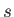particle: that is, a particle for which the eigenvalue of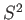is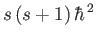. Here,is either an integer, or a half-integer. The eigenvalues of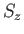are written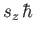, where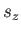is allowed to take the values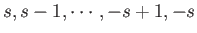. In fact, there are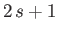distinct allowed values of. Not surprisingly, we can represent the state of the particle bydifferent wavefunctions, denoted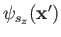. Here,specifies the probability density for observing the particle at position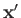with spin angular momentumin the-direction. More exactly,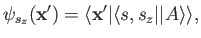(5.111)

where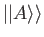denotes a state ket in the product of the position and spin spaces. The state of the particle can be represented more succinctly by a spinor-wavefunction,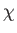, which is simply thecomponent column vector of the. Thus, a spin one-half particle is represented by a two-component spinor-wavefunction, a spin one particle by a three-component spinor-wavefunction, a spin three-halves particle by a four-component spinor-wavefunction, and so on.

In this extended Schrödinger/Pauli scheme, position space operators take the form of diagonal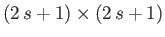matrix differential operators. Thus, we can represent the momentum operators as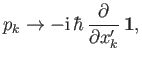(5.112)

where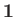is theunit matrix. [See Equation (5.91).] We represent the spin operators as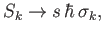(5.113)

where theextended Pauli matrix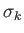(which is, of course, Hermitian) has elements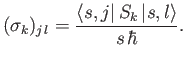(5.114)

Here,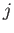,are integers, or half-integers, lying in the range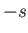to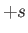. But, how can we evaluate the brackets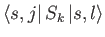and, thereby, construct the extended Pauli matrices? In fact, it is trivial to construct the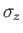matrix. By definition,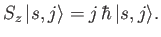(5.115)

Hence,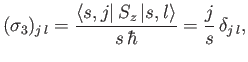(5.116)

where use has been made of the orthonormality property of the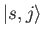. Thus,is the suitably normalized diagonal matrix of the eigenvalues of. The elements of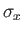and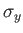are most easily obtained by considering the ladder operators,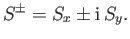(5.117)

We know, from Equations (4.55)-(4.56), that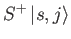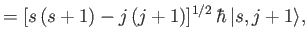(5.118)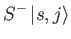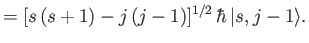(5.119)

It follows from Equations (5.114), and (5.117)-(5.119), that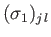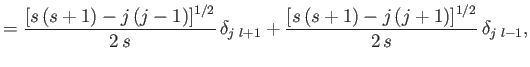(5.120)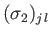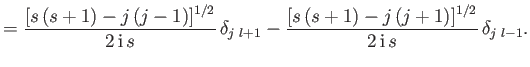(5.121)

According to Equations (5.116) and (5.120)-(5.121), the Pauli matrices for a spin one-half (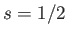) particle (e.g., an electron, a proton, or a neutron) are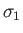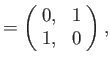(5.122)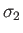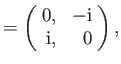(5.123)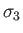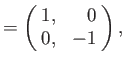(5.124)

as we have seen previously. For a spin one (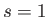) particle (e.g., a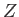-boson or a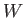-boson), we find that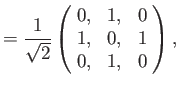(5.125)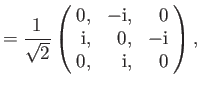(5.126)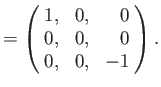(5.127)

In fact, we can now construct the Pauli matrices for a particle of arbitrary spin. This means that we can convert the general energy eigenvalue problem for a spin-particle, where the Hamiltonian is some function of position and spin operators, intocoupled partial differential equations involving thewavefunctions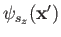. Unfortunately, such a system of equations is generally too complicated to solve exactly.Next: Exercises Up: Spin Angular Momentum Previous: Factorization of Spinor-Wavefunctions
Richard Fitzpatrick 2016-01-22